# Quantisation of Poles in the Z-plane

Back in this posting, I talked about biquads and their use in digital signal processing for making linear filters (what most of us call “equalisers”). Part of that explanation showed that a biquad is formed of a feed-forward section and a feed-back section, and you could swap the order of these two and get the same results. In a “Direct Form 1” implementation, the feed-forward comes first, as shown in Figure 1.

in the “Direct Form 2” implementation, the feed-back comes first, as shown in Figure 2.

There are advantages and disadvantages to each of these implementations, depending on things like how the rest of your system is implemented, and what, exactly you’re expecting the biquad to do.

However, for this posting, we’re going to “zoom in” a bit to the feed-back portion of the above diagrams. This portion of the biquad provides the “poles” in the Z-plane, as I described in the “Intuitive Z-plane” series of postings last week.

If I separate the feed-back portion of the above figures, it would look like Figure 3:

The locations of the poles (and therefore the magnitude response) of this portion of the filter are dependent on the gains at the outputs of the two 1-sample delays. What happens when these gains do not have an infinite resolution (which they can’t, because everything in a digitally-represented world is quantised to a finite number of steps)?

Before we go any further with this, I have to put in a reminder that quantisation (or “rounding”) in a DSP world is done in binary – not decimal. So, the quantisation that I’m about to do isn’t the same as stopping a couple of digits after the decimal…

In a world with infinite resolution, I can set the values of those two gains to be anything I want, and therefore the poles in my system can be anywhere within the circle. (We’ll assume that we don’t want a pole on the circle because that would result in a gain of ∞ dB, which is very loud.) However, let’s say that we quantise the gain values to a 4-bit binary value, where the first bit is reserved for the +/- indicator. This means that we only have +/- 2^3 possibilities, or 8 values, one of which is +0, and another is -0 (which is the same thing…). So, in other words, we have 7 possible negative values, 7 possible positive values, and 0.

The end result of this is that the poles have a limited number of locations where they can be placed. For the 4-bit quantisation described above, the resulting locations look like Figure 4.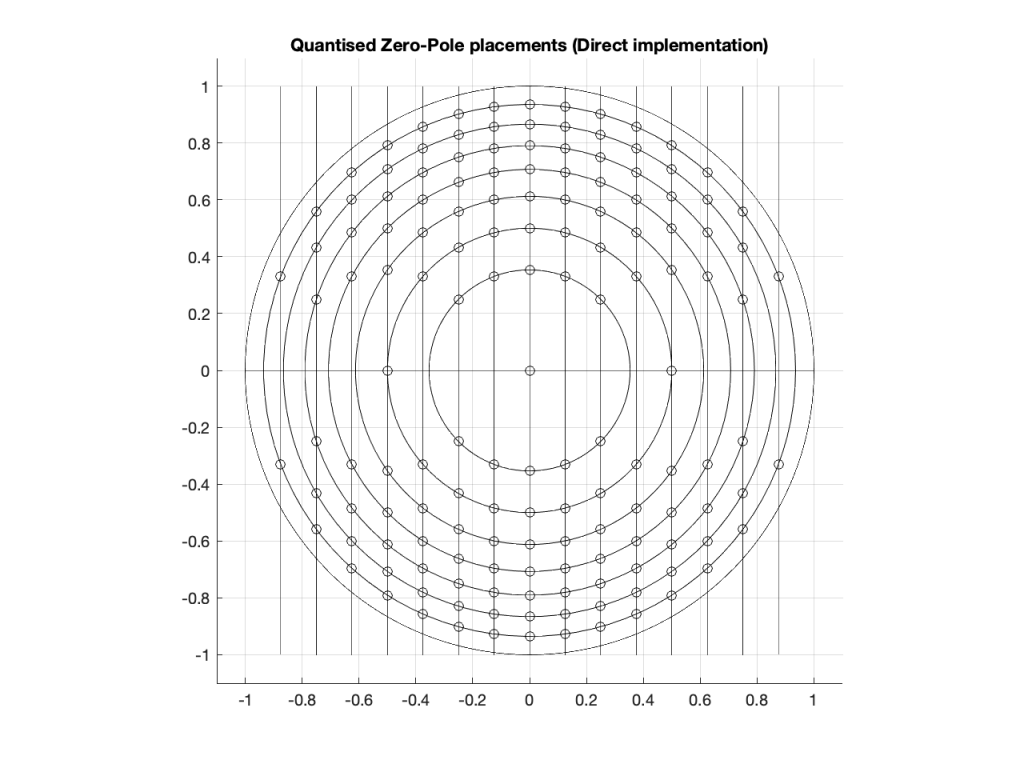Figure 4: The circles indicate the possible locations of the poles as a result of quantisation of the gain coefficients in a 4-bit Direct Form implementation.

Remember that we’re not talking about quantisation of the audio signal – it’s quantisation of the gain coefficients inside the biquad that will have an impact on the response of the filter.

Of course, it would be crazy to implement a biquad using only 4-bit quantisation for the gain coefficients. However, the point of this posting is not to show that biquads suck. It’s only to show one possibly important aspect of them if you’re a DSP engineer – but we’re getting there.

Just for fun, let’s increase the resolution of the system to 6 bits: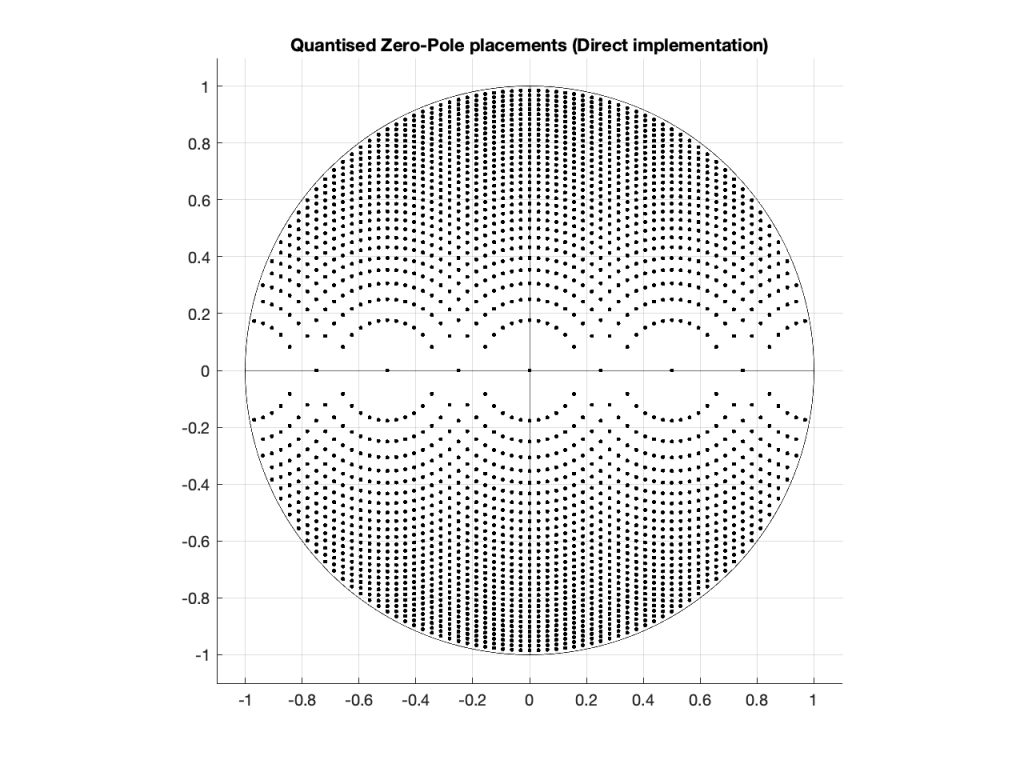Figure 5: The dots indicate the possible locations of the poles as a result of quantisation of the gain coefficients in a 6-bit Direct Form implementation.

… or to 8 bits: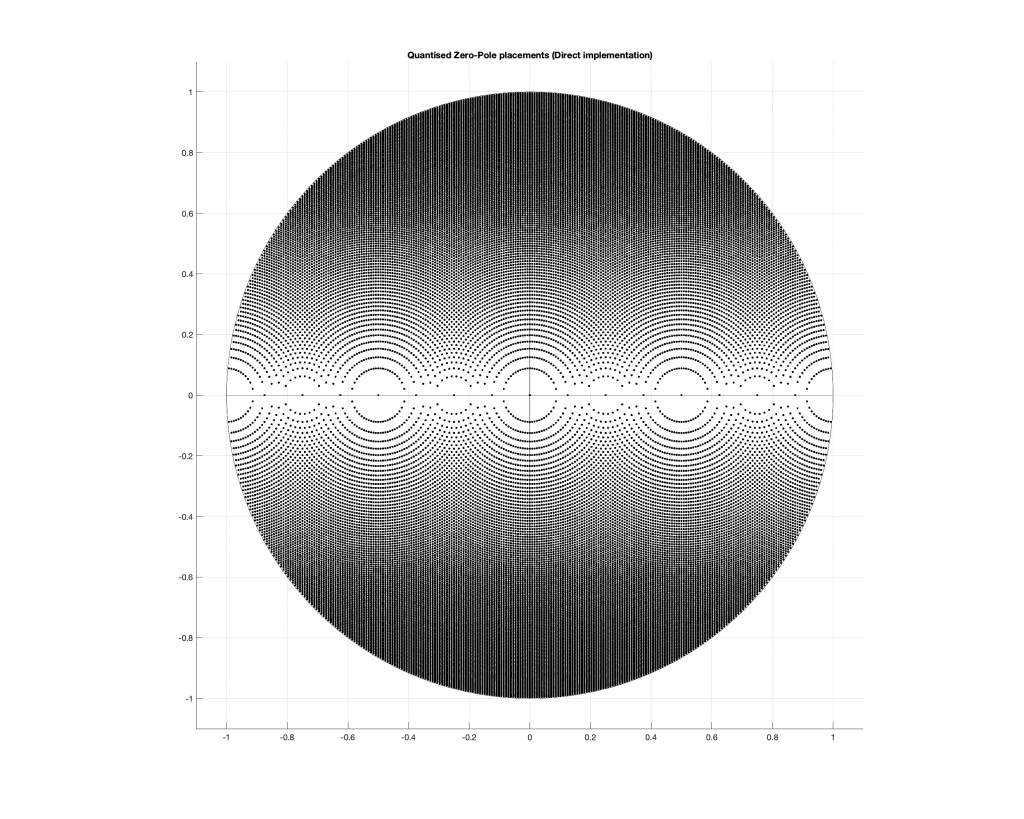Figure 6: The dots indicate the possible locations of the poles as a result of quantisation of the gain coefficients in a 4-bit Direct Form implementation.

Any higher than this and the pattern will just get so dense that it’ll turn black, so I’ll stop.

## So what?

At this point, you may be asking “so what?” The answer to this very important question lies on the far right side of all of those graphs. Notice that there aren’t any locations near the point on the graph where X=1 and Y=0. You may remember from Figure 7 in this posting that I did last week, that that’s the point on the Z-plane that corresponds to very low frequencies.

This means that, if you want to create a digital filter, and you need a pole near 0 Hz, you’re going to run into some trouble with a Direct Form implementation of the biquad. Yes, the higher the bit depth of the gain coefficients, the closer you can get, but this might not be the best way to do things.

There is another option for implementing your feed-back portion of the biquad. You can use a design called a Coupled Form instead, which is shown in Figure 7 (compare it to Figure 3).

Notice that you still have two 1-sample delays, however there are now 4 gains instead of 2. How can this be better? Well, in a system with infinite resolution on the gain coefficients, it’s not. Given the appropriate choice of gain values, this implementation will do exactly the same thing as the Direct Form implementation if your resolution is infinite.

However, if you have a limited resolution, then the available locations of the poles on the Z-plane are very different. Let’s use the 4-bit, 6-bit, and 8-bit quantisations of the gain values again: these are shown in Figures 8 to 10.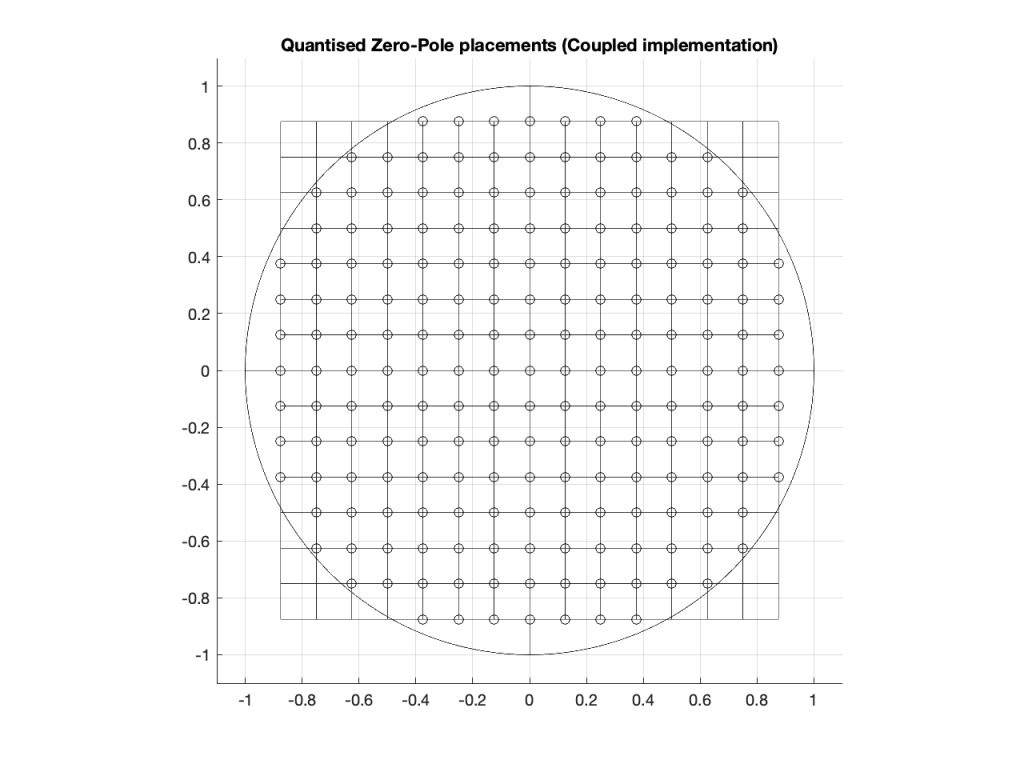Figure 8: The circles indicate the possible locations of the poles as a result of quantisation of the gain coefficients in a 4-bit Coupled Form implementation.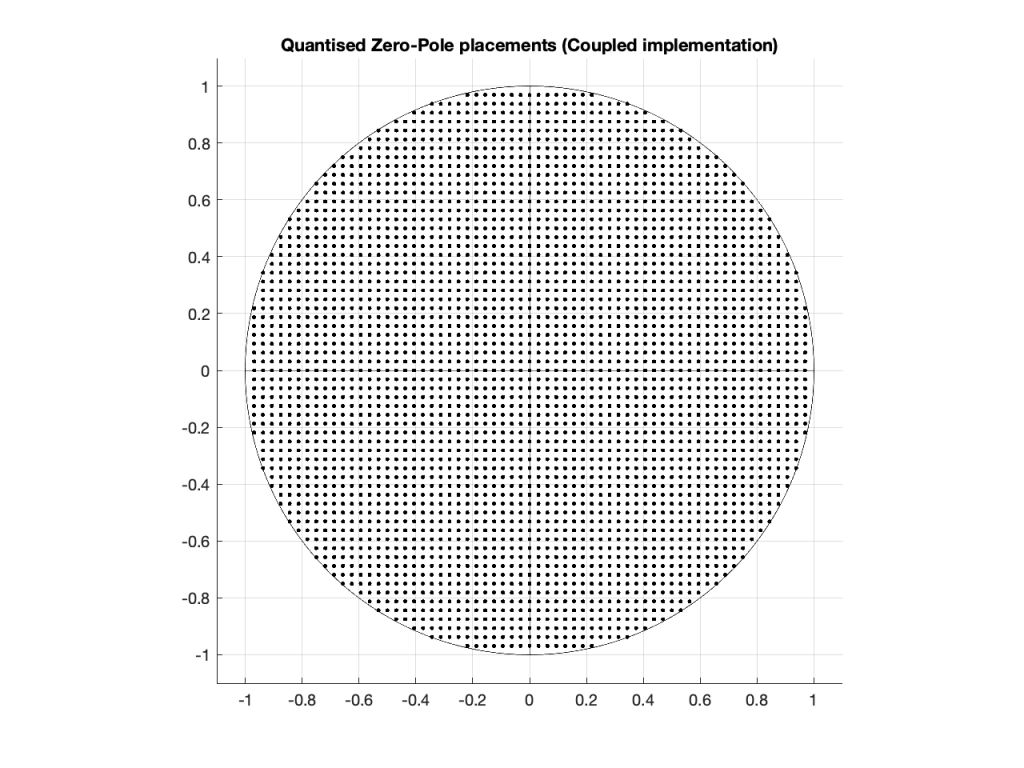Figure 9: The dots indicate the possible locations of the poles as a result of quantisation of the gain coefficients in a 6-bit Coupled Form implementation.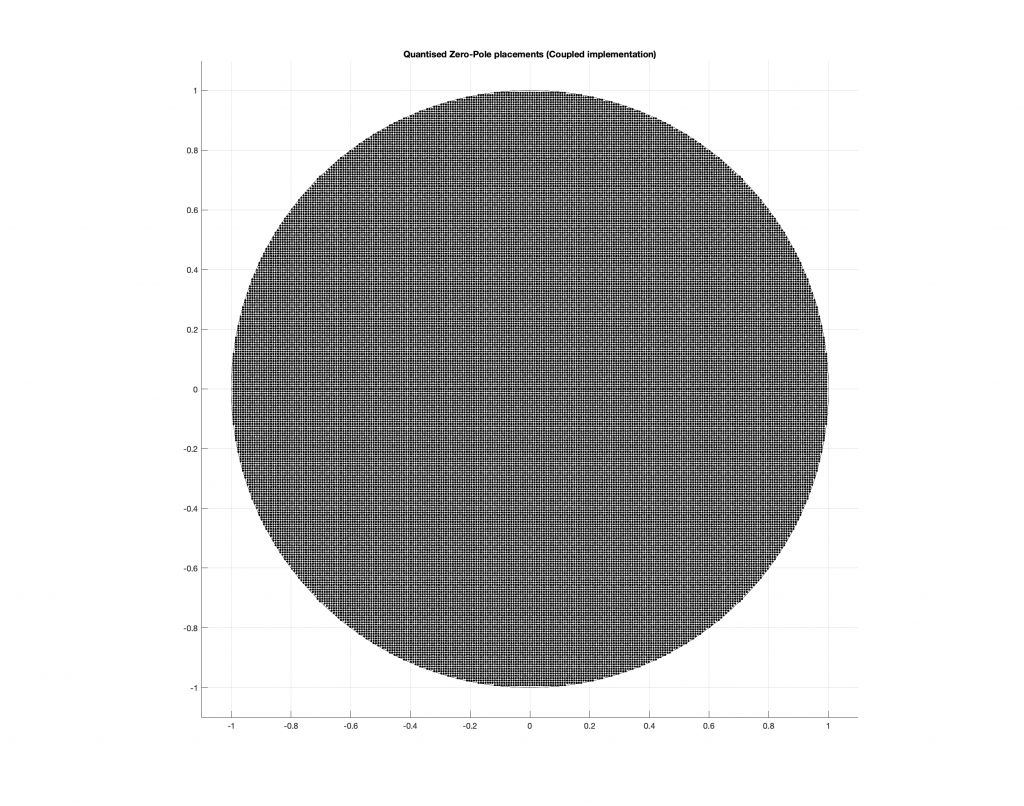Figure 10: The dots indicate the possible locations of the poles as a result of quantisation of the gain coefficients in a 8-bit Direct Form implementation.

As you can see in Figures 8 through 10, the Coupled Form implementation, given the same resolution on the gain coefficients, will give you much better placement opportunities for the poles in the low frequency region than the Direct Form implementation.

Of course, in all of these examples, I’m only showing up to an 8-bit word, and a typical DSP runs uses a lot more than 8 bits for the gain coefficients. So, it’s possible that, in the real world, the actual resolution is high enough that this is of no concern whatsoever.

However, if you’re building a very-low-frequency filter an if the magnitude response isn’t exactly what you’re looking for, this might help you get a little closer to your goal.

It’s important to point out here that the quantisation of the locations of the zeros is not the same as this. Someday, I’ll come back to this and plot the expected vs. actual magnitude responses of some biquads where I’ve quantised the zeros and poles, just to see how badly things go wrong when they do…